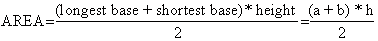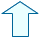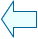GeomEtric sHAPES ON THE PlanE_4 Section: Geometry

1. THE AREA OF A TRAPEZIUM

The formula to find the area of a trapezium is1.- Click on the next_step control button and try to understand all the steps.

2.- Note that trapezium ABCD can be divided into two triangles and so we can work out the formula we want by using the formula to find the area of a triangle.

3.- Carry out the same steps in your exercise book with a pencil and piece of paper.

 Move the vertices of the trapezium to see more examplesAgustín Muñoz NúñezSpanish Ministry of Education. Year 2001